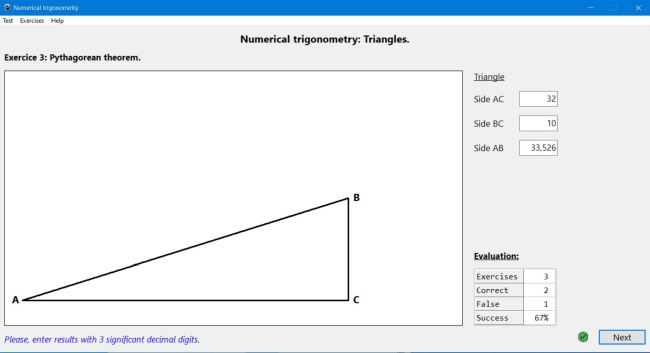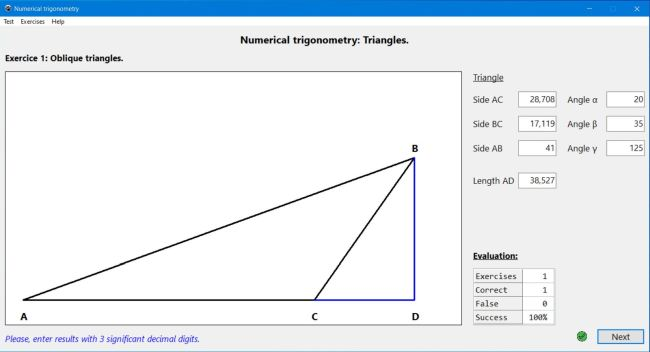# Computing: Free Pascal Programming

## Numerical trigonometry: Triangles.

Description: Mathematics trainer (exercise generator), concerning numerical trigonometry, or more precisely triangles. 5 types of exercises are considered:

• The Pythagorean theorem.
• Similar right triangles.
• Trigonometric ratios.
• Oblique triangles.
• The law of sines.
The application draws the triangle(s) and gives some of the values (e.g. the lengths of 2 sides, with the Pythagorean theorem) and the user has to calculate the other one(s), the different exercise types being individually selectable, so the students can test their knowledge for specific topics.

Possible extensions: Exercises, mixing the different types of calculations.

Free Pascal features: Showing/hiding/modifying controls, depending on actual situation. Drawing geometrical shapes, using canvas.

Screenshots: